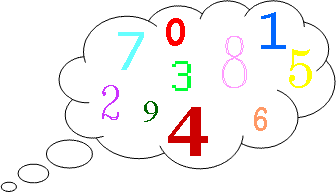Here is an alternative version of the “Think of a Number” trick.

Think of two whole numbers under 10.
Take one of them and add 1.
Multiply by 5.
Subtract 1.
Double again.
Subtract 8.
Halve this number and tell me your answer.

I can work out both your numbers very quickly. How?

Choose some different pairs of numbers and repeat the process.
Can you find a good explanation of how the trick works?

This problem is adapted from the NRICH task Think of Two Numbers  with permission of the University of Cambridge. All rights reserved.

Tagged with: